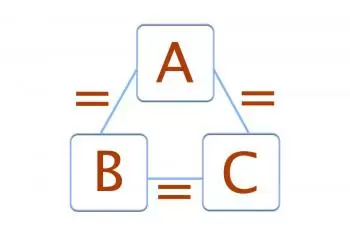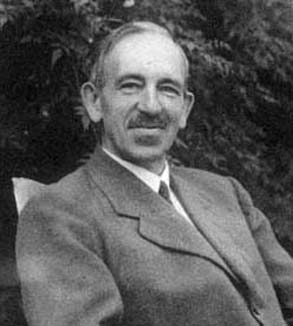# Zeroth Law Of ThermodynamicsThe zeroth law of thermodynamics states that "if two systems are in thermal equilibrium with a third, they are also in equilibrium with each other." It is also known as the zeroth principle of thermodynamics.

If A is in equilibrium with B and A is also in thermal equilibrium with C, we can conclude that B is in thermal equilibrium with C.

Two bodies are said to be in thermal equilibrium when their state variables do not change upon contact. Around this simple idea the zeroth law is established.

Curiously, the zeroth law was the last of the laws of thermodynamics to be introduced. After verifying that heat is one form of energy that could be transformed into another, the terminology became known as thermodynamics.

To obtain a logical structure in the presentation of thermodynamics, it was necessary to place another law before those that had been previously stated. Thus, that other law was called the zeroth law of thermodynamics.

With the foundations of this law we can find out if two bodies X and Y are in equilibrium. To do this, simply check if the two bodies have the same temperature using a thermometer.

## Statement of the zeroth law of thermodynamics

The statement of the zeroth law of thermodynamics says that: "Two systems in thermal equilibrium with a third are in equilibrium with each other."

The zeroth law of thermodynamics allows us to differentiate bodies from each other with respect to their "degree of heating." We identify this attribute, which is a property of the system, with its temperature, which turns out to be a macroscopic concept (measurable).

Through these concepts we can understand the operation of devices called thermometers. Thermometers are devices that measure the temperature of bodies.

It should be noted that the formulation of the zeroth law of thermodynamics contains three firm ideas:

• The existence of a state variable, called temperature.

• Equality of temperatures as a condition for thermal equilibrium between two systems, or between parts of the same system.

• The existence of a relationship between the independent variables of the system and the temperature, called the equation of state.

## Examples of the zeroth law of thermodynamics

### Ice and water

In this example, we put an ice cube and a glass filled with hot water in an airtight box. Three elements appear in this experiment: ice, water, and the air inside the box.Initially, ice has a lower temperature than air. So the air will give energy to the ice.

The water is at a higher temperature than the air. In these conditions the water will transfer energy to the air.

After a while, the ice will have the same temperature as air. Water will also have the same temperature as air. Both elements will be in thermal equilibrium with air. Therefore, according to the 0 principle of thermodynamics, water and ice (which now is already melted) will have the same temperature.

### Thermometer example

Another example that will help you better understand the concept of thermal equilibrium is that of the thermometer.

Consider a body B consisting of a tube with a capillary containing mercury. Its height levels above the capillary represent different temperatures.

Now consider a body A. For example the human body. If you bring the thermometer close to the body and leave it long enough, the thermometer will reach the value corresponding to body temperature.

The thermometer and the human body will be in thermal equilibrium with each other. Therefore, they will have the same numerical value for the temperature property.

## Concepts for understanding the zeroth principle

To understand the zeroth law of thermodynamics, consider the behavior of two or more systems constituted as follows.

Systems A and B are separated from each other by an adiabatic wall. An adiabatic wall is a wall that does not allow heat exchange.

Each of the two points is in thermal contact with the third system C through diathermic walls. These walls allow the exchange of thermal energy. The whole complex is surrounded by an adiabatic wall.

Our experience says that both systems will achieve thermal equilibrium with the third. Furthermore, no subsequent change will take place if the adiabatic wall separating A and B is replaced by a diathermic wall.

## History of the zeroth law of thermodynamics

The ideas of "hot" and "cold" have always been part of man's sensory experiences.

Two of the first scientists to express these ideas were Leonardo Da Vinci and Galileo. These scientists knew that upon contact with a third body, usually air, two or more bodies in contact with it "mixed in an appropriate way until reaching the same condition."

This condition was achieved due to the tendency of hot bodies to diffuse their energy to colder bodies. This flow of energy is called heat or heat energy. Thus, we can perceive the tendency of heat to diffuse from any hot body to cooler ones in its surroundings.

This heat transfer takes place until none of the bodies in the system is able to absorb more heat.The zeroth law of thermodynamics has this name thanks to Ralph H. Fowler (1889-1944), a great English physicist. In the 20th century, after a long time of development of the experimental law, he considered it as a basic law.

Without this law the concept of temperature could not be defined and it was postulated that: "If two bodies are in thermal equilibrium with a third, they will be in thermal equilibrium with each other".

However, it became necessary to structure the presentation of thermodynamics in a more logical way. Because the first and second laws had already been formulated, then the term zeroth law came up.

Since then this denomination has been used in physics.

## Summary

The zeroth law of thermodynamics states that two elements that are in thermal equilibrium with a third element, the two initial elements are also in thermal equilibrium with each other.

Author:

Published: June 29, 2016
Last review: May 31, 2020